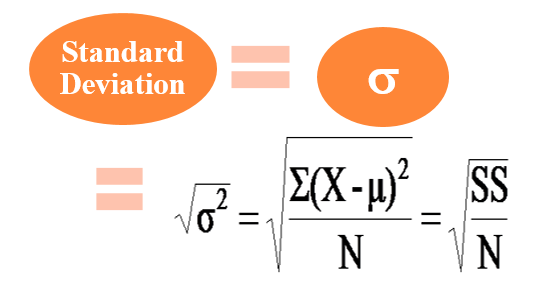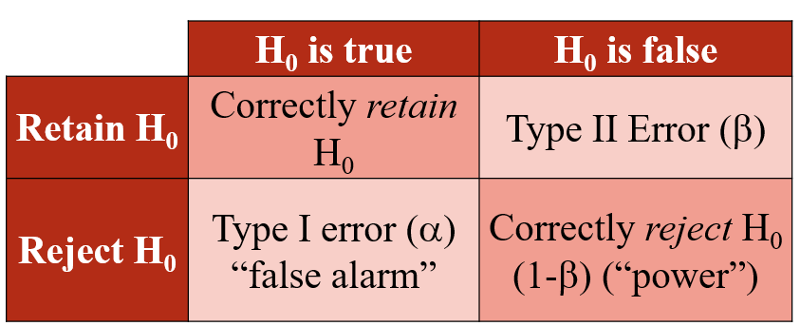##### Psych 311 Unit 3 study guide

0
Set Details Share
created 5 years ago by wouhib
22 views
updated 5 years ago by wouhib
Page to share:
Embed this setcancel
COPY
code changes based on your size selection
Size:
X
1

Descriptive vs. inferential statistics, statistic vs. parameter

- Descriptive statistics: A “description” or summary of a set of data, organizing a set of scores into a graph or a table, calculating average score

- Inferential statistics: A way to use the data from a sample (as in a study) to answer questions about a population, generalizing from your study, to a larger group

- Statistic: A summary value that describes a sample

- Parameter: A summary value that describes a population

2

...

A frequency distribution is a graph of score value (X-axis) against frequency (Y-axis)

Can give rough ideas of: variability (how spread out the scores are), central tendency (the "middle" of the distribution), shape of distribution

Histograms: Bars are drawn over ranges of scores (not individual scores), Require continuous variables (that’s why bars are drawn touching), can't see individual scores

Stem-and-leaf: Not seen as frequently, but can be useful, Stem-and-leaf creates “bars” made up of the raw scores

Bar Graphs/Pie charts: Good for categorical variables, sometimes harder for people to interpret

Normal distributions: A normal distribution refers to a family of distributions that share common properties, technically, these are distributions with very large populations (or theoretical)

- know symbols for population/sample mean and standard deviation

3

Distributions: Ability to interpret/understand frequency distributions, types of distributions (e.g., symmetrical, skewed positive, skewed negative), tails, characteristics of a normal distribution, area under the curve

Normal distributions are always symmetric, most of the scores are in the middle, centered around the mean, fewer scores in the “tails” (ends) of the distribution, tails are mirror images of each other

Positive skew: sometimes called “right-skew”, when most of the scores are lower, but some are high (the tail “points” to the right). Examples: Income, depression, opiate use, aggression

Negative skew: sometimes called “left-skew”, when most of the scores are high, but a few are low. Examples: Exam scores, Optimism, Self-Worth

Area under the curve: The area under the curve is a function of μ (or x̅) and σ (or s), 50% of the curve is above the mean, normal curves can all be “partitioned” as a function of mean and SD values. This partitioning can be very precise.

4

Central tendency: definitions/descriptions of mean, median, and mode; circumstances under which each measure of central tendency can/should be used, strengths and limitations of each, and how to calculate/find each

Mean: A set of scores computed by summing all of the scores of interest, and dividing by the total number of scores.

- Population μ, sample M or x̅

- Adding, subtracting, multiplying or dividing a constant from each score in the distribution is the same as adding, subtracting, multiplying or dividing that same constant to/from the mean.

- Weakness: it is sensitive to extreme scores.

Median: The 50th percentile, it is the point below which exactly half of the scores lie.

- Less sensitive to one or two “extreme” scores (in contrast to mean).

- Weakness: simply not “as good” as the mean for statistical inference

Mode: The score (or category) with the greatest frequency (the score/category that occurs most often in the distribution)

- Only measure of central tendency that can be used with categorical data

- Weaknesses: it is possible to have no mode or more than one mode, problems with inference to the population

5

Variance and standard deviationVariability: Difference between an individual score (Xi) and the mean of the distribution (μ), (Xi - μ)

Sum of squares (SS): Cannot compute an “average” deviation because ∑(Xi - μ) = 0 (always) therefore square each deviation score prior to summing which ensures all values ≥ 0

Variance: SS is affected purely by number of scores thefore “Sum” score increases as N increases but dividing by N adjusts for sample size

Standard deviation: Variance represents the average squared difference from the mean”(“average squared deviation”), to obtain “typical deviation” (i.e., standard deviation), calculate the square root of the variance

• Sensitive to all scores
• Adding (or subtracting) a constant to each score does NOT change the SD
• Multiplying (or dividing) each score by a constant results in the SD being multiplied by the same constant.

Sample variance: If sample SS is divided by sample size (n) to calculate variance, the value will be too small. Rather than use “n” when calculating sample variance, (n-1) is used, which is degrees of freedom.

6

Hypothesis testing

Scientific hypothesis: The “prose” hypothesis, In words, what the researcher expects to find. Example:

- A new type of therapy will be more effective at reducing depressive symptoms than the old type.

Null hypothesis (H0): The hypothesis of NO effect or NO relation between variables

Alternative (H1): Hypothesis of “effect” which includes, but may not be limited to, what the researcher expects to find

Inferences in hypothesis testing

• If probability the statistic came from the sampling distribution is low (e.g., p< .05), reject our initial assumption that H0 is true (i.e., reject the null)
• If probability the statistic came from the sampling distribution is reasonable (e.g., p> .05), retain our initial assumption that H0 is true (i.e., retain the null)
7

Errors in hypothesis testingType I error rate (α)

• Probability of rejecting H0 when H0 is true.
• Probability of a is set by researcher.
• Traditionally, a is set at either .05 or .01.

Type II error rate (β)

• Probability of retaining H0 when H0 is false.
• “Missing” the true effect.

Decreasing type II error

• Larger values of a are used
• A one-tailed test is appropriately used
• Larger samples are used
• The size of the effect is increased
• The standard deviation in the population is decreased

Increasing alpha: Decreases Type II error (if H0 is false), increases probability of type I error (if H0 is true)

Relationship between type I error and type II error: